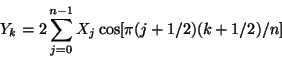Next: , Previous: , Up: What FFTW Really Computes   [Contents][Index]

#### 4.8.3 1d Real-even DFTs (DCTs)

The Real-even symmetry DFTs in FFTW are exactly equivalent to the unnormalized forward (and backward) DFTs as defined above, where the input array X of length N is purely real and is also even symmetry. In this case, the output array is likewise real and even symmetry.

For the case of `REDFT00`, this even symmetry means that Xj = XN-j, where we take X to be periodic so that XN = X0. Because of this redundancy, only the first n real numbers are actually stored, where N = 2(n-1).

The proper definition of even symmetry for `REDFT10`, `REDFT01`, and `REDFT11` transforms is somewhat more intricate because of the shifts by 1/2 of the input and/or output, although the corresponding boundary conditions are given in Real even/odd DFTs (cosine/sine transforms). Because of the even symmetry, however, the sine terms in the DFT all cancel and the remaining cosine terms are written explicitly below. This formulation often leads people to call such a transform a discrete cosine transform (DCT), although it is really just a special case of the DFT.

In each of the definitions below, we transform a real array X of length n to a real array Y of length n:

#### REDFT00 (DCT-I)

An `REDFT00` transform (type-I DCT) in FFTW is defined by:.
Note that this transform is not defined for n=1. For n=2, the summation term above is dropped as you might expect.

#### REDFT10 (DCT-II)

An `REDFT10` transform (type-II DCT, sometimes called “the” DCT) in FFTW is defined by:.

#### REDFT01 (DCT-III)

An `REDFT01` transform (type-III DCT) in FFTW is defined by:.
In the case of n=1, this reduces to Y0 = X0. Up to a scale factor (see below), this is the inverse of `REDFT10` (“the” DCT), and so the `REDFT01` (DCT-III) is sometimes called the “IDCT”.

#### REDFT11 (DCT-IV)

An `REDFT11` transform (type-IV DCT) in FFTW is defined by:.

#### Inverses and Normalization

These definitions correspond directly to the unnormalized DFTs used elsewhere in FFTW (hence the factors of 2 in front of the summations). The unnormalized inverse of `REDFT00` is `REDFT00`, of `REDFT10` is `REDFT01` and vice versa, and of `REDFT11` is `REDFT11`. Each unnormalized inverse results in the original array multiplied by N, where N is the logical DFT size. For `REDFT00`, N=2(n-1) (note that n=1 is not defined); otherwise, N=2n.

In defining the discrete cosine transform, some authors also include additional factors of √2 (or its inverse) multiplying selected inputs and/or outputs. This is a mostly cosmetic change that makes the transform orthogonal, but sacrifices the direct equivalence to a symmetric DFT.

Next: , Previous: , Up: What FFTW Really Computes   [Contents][Index]